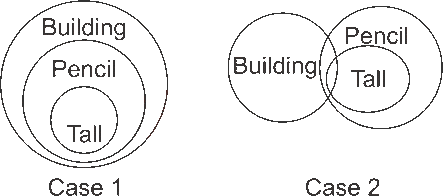Question of The Day22-09-2022

Below are two statements. You have to take the given statements to be true even if they seem to be at variance with the commonly known facts.

Statements:

I. Some buildings are tall.

II. All tall are pencils.

Decide which of the below conclusions logically follows from the given statements.

A. All pencils can be building

B. All pencils are tall

C. Some tall are not pencil

D. No pencil is tall

Correct Answer : b ) A

Explanation :

According to question:

Given statements can be depicted by the following possible cases,Therefore, from the above venn diagrams we can say that,

From case 1 we can say that possibility given in Conclusion A is true and thus conclusion A follows the given statements.

Conclusion B is not possible

Conclusion C is not possible

Conclusion D is not possible

Hence, (b) is the correct answer.0